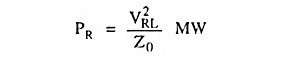## Performance of Long Transmission Lines Interview Questions and Answers:

1. What is the range of surge impedance for an overhead transmission line ?

Ans. 400-600 Ω.

2. What is the range of surge impedance in case of underground cables ?

Ans. 40-60 Ω.

The ‘natural power’ or ‘surge impedance loading’ of a transmission line is given aswhere VRL is the receiving-end voltage in kV and Z0 is the surge impedance in ohm.

The above expression gives a limit of the maximum power that can be delivered by a line and is useful in the design of transmission lines. This can be used for the comparison of loads that can he carried on the transmission lines at different voltages.

4. How does synchronous phase modifier differ from a synchronous motor ?

Ans. Synchronous phase modifier differs from an ordinary synchronous motor in as much as they are built for the highest economical speeds, and provided with smaller shafts and bearings and special attention is paid for securing a high overall efficiency.

5. How does the reactive power of a transmission line vary for given values of VS, VR and X ?

Ans. For fixed values of VS, VR and X, the reactive power is proportional to the magnitude of voltage drop in the line (i.e., VS – VR).

6. Why high and extra voltages are adopted for transmission of bulk power over long distances ?

Ans. This is because the maximum power transferred over a given line increases with the increase in VS and VR.

7. What is meant by receiving-end power circle diagram ?

Ans. The circle diagram drawn with receiving-end true and reactive power components as the horizontal and vertical ordinates is called the receiving-end power circle diagram.

8. What is meant by sending-end power circle diagram ?

Ans. The circle diagram drawn with sending-end true and reactive power components as the horizontal and vertical ordinates is called the sending-end power circle diagram.

9. What is universal power circle diagram ?

Ans. In the ordinary receiving-end power circle diagram, if the receiving-end voltage is kept constant, the centre of the circles is fixed and for different sending-end voltages circles are drawn; these will be concentric but if the receiving-end voltage is not constant the circles will not be concentric. Similar is the case with the sending-end power circle diagram. When sending-end voltage is not constant, its centre will also vary.

To overcome the above limitation the universal power circle diagram is drawn. In this diagram each distance on the original diagram is divided by V2/B where V is the “reference or base voltage” and B is the generalized circuit constant. This gives dimensionless units.

Scroll to Top